﻿ The Credit Portfolio Management by Structural Models: A Theoretical AnalysisPublications are Open
Access in this journal
Article Versions
Export Article
• Normal Style
• MLA Style
• APA Style
• Chicago Style
Review Article
Open Access Peer-reviewed

The Credit Portfolio Management by Structural Models: A Theoretical Analysis

International Journal of Global Energy Markets and Finance. 2018, 1(1), 11-20. DOI: 10.12691/ijgefm-1-1-3
Published online: April 24, 2018

Abstract

The purpose of this paper is to study the credit portfolio management by the structural models (Moody's KMV model and Credit Metrics model) also defined by the models of the value of the firm. The development of this type of models is based on a theoretical basis developed by several researchers. The evolution of their default frequencies and the size of the loan portfolio are expressed as functions of macroeconomic and microeconomic conditions as well as unobservable credit risk factors, which explained by other factors. We develop two sections to explain the different characteristics of those two models. The purpose of all its models is to express the default probability of credit portfolio.

1. Introduction

The problem of evaluation of the failure probability of any borrower was the center of the bankers as soon as they began to lend some money. The quantitative modeling of the credit risk for a debtor is rather recent in fact. Besides, the modeling of the credit risk associated with instruments of a portfolio of credit such as, the loans, the pledges, the guarantees and the by-products (who constitute a recent concept).

A certain number of models were developed, including at the same time the applications of property developed for the internal custom by the financial institutions, and the applications intended for the sale or for the distribution 1.

The big financial institutions recognize his necessity, but there is a variety of approaches and rival methods. There are two types of models of credit portfolio in the course of use at present 2:

• Moody's KMV model (Portfolio Model).

• CreditMetrics model by JPMorgan.

The main idea for this study is to answer the question follows: How the default probability is defined by the credit portfolio models?

Then, the organization of this paper is as follows. In section 2, we present the fundamental of structural models and we define the forces and the weaknesses of each model. We provide the presentation of the Moody's KMV model in section 3. The section 4 is considered to present the development of the CreditMetrics model by JPMorgan. Section 5 concludes.

2. The Structural Models

The structural models of management of credit portfolio were presented by Merton 3 and then, developed by Leland 4, Leland and Toft 5, Anderson and Sundaresan 6 and Jarrow 7. The characteristics to define a structural model are given by two conditions:

• The process of management of the assets of the company has to be known on the market in which this one operates.

• The structure of the liabilities of the company has to be known by all the actors operating on the market of this one.

In the practice, to examine the models of management of credit portfolio, it is necessary to employ parameters estimated implicitly because the values of the assets of the company are not observable. Nevertheless, the majority of the empirical evidence does not retain the structural models. The implicit prices obtained from the structural models does not seem to match the structure of maturity of the efficiencies on the assets of the company 7, 8, 9, 10 and to allow the forecasts of defect of the borrowers.

The analysis of the model of Merton 3 finds that this one supposes that the value of the firm follows a process of distribution and the defect occurs when the value of the firm falls below the nominal value of the debt on the date of maturity of this one. In this respect, this model serves to determine a threshold of defect.

The development of Merton's model is made by adding the other variables such as; the interest rate, the optimal permanent capital, the variable time of the threshold of default, the unfinished accounting information and the risk of the events of defect.

The structural models are based on the theory of the options and the structure of the capital of the company 11. In this aligned, the bankruptcy of a company took place when the value of assets is situated below the value of its debt. The structural models or the models of the value of the firm are based on the approach of Merton 3 which supposes that the failure of a company appears in case the market value of its assets is lower than a certain threshold of its debts.

Generally, the models of credit portfolio management resting on the approach of Merton are the model KMV (Kealhofer, McQuown and Vasicek) of Moody and the CreditMetrics model of JPMorgan (1997). The distinction between both structural models was described in Table 1.

The structural models are also called models of the asset volatility. The Structural aspect of the models comes because there is a historical story behind by default that is something manages to start by default. The structural models are rooted in the knowledge of Merton. In Merton's model, the correlation of defect has to be a function of correlation of assets. The estimation of a structural model requires the implementation of the market value of the assets of the company and its volatility.

In the practice, the value of assets and their volatility are not observable for the most part of companies. The structural models lean strongly on the existence of assets quoted on the stock exchange so that we can estimate the necessary parameters.

3. The KMV Model

The KMV model of credit portfolio management was elaborated for the first time in 1993. This model allowed the development of several models of quantification of the credit risk: Credit Monitor, Credit Edge and Private Firm Model for the individual credit risk and Portfolio Manager for the credit risk of a portfolio.

The model KMV rests bases on the notion of default distance which is calculated by basing itself on the barrier which engages the defect. As soon as, the distance in the defect is calculated, it transformed into the probability of failure (Expected Default Frequency: EDF).

The KMV model which was developed by the Moody’s-KMV company is based on the theory of the prices of Merton options. It is about an abstract frame used to estimate the default probability of a company. The KMV model supposes that the company is in situation of defect when the value of its asset is less than the value of its debts. The Figure 1 explains the relation among the estimated own capital and the value of the asset. According to Merton's basic idea, in the KMV model the value of the own capital of the company is considered as being an option to buy. So, the value of the asset is considered as being the underlying asset and the debt represents the price of exercise 12.

• Figure 1. The relation between the market value of the assets of the company and the value of the debt 

In Figure 1, VA indicates the initial investment of the shareholders of the company; X indicates the point of default which corresponds to the sum of the long-term debt and half of the current liabilities. When the value of assets (VA) is superior to the debt (X), the shareholders will choose to gain profits staying after payment of the debts (VA - X) and these will be chosen by default, what is shaped with a net value raised in the Figure 1. In this case, the investor executes the option to buy.

So, if the value of assets is lower than the debt (VA < X), the shareholders will choose by default the transfer of the active total for the benefit of the creditors, what is coherent with a constant value of own capital indicated in the Figure 1, and it means that the option to buy is not executed.

Generally speaking, the shareholders receive Max (VA - X, 0) in the date of maturity T. According to Merton's model, the evolution of the market value of the assets of the company follows a process of geometrical distribution of the following shape:Where,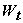denotes the process of Wiener Standard is,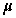is the average of the efficiency of assets andis the standard deviation of the efficiency on assets. The market value of the company is given by basing itself on the purchase price of a European option to buy supplies by Black and Scholes 13.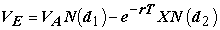Where, N(.) indicate the function of distribution of the normal law with 14:In the KMV model, there is a hypothesis which rests on the structure of the capital of the company. So, this capital has to consist only by actions, current liabilities and in the long term and convertible prices. Really, the value of the company VA and the volatility of assetsare not observable 12. We are going to deduct these two values by using the values of the options VE.

So land us note that: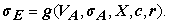Where, c is the coupon paid on the long-term debt, r is the interest rate without the risk andis the volatility of share prices.

By applying the Lemma of Itô to these two functions and by arranging the terms we obtain: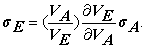With: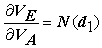who is deducted from the equation which measures the value of the VE which is defined by the following expression: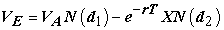Thus,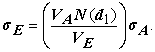Further to this transformation, we obtain a system of equation to two unknownsand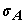: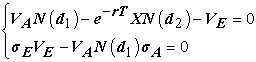If the expressions ofandare determined, then, we can arrive at the writing of the following formulation of the distance of defect (DD):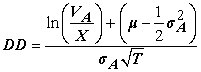According to the KMV model the distance of defect is defined in the following way: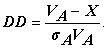From the distance of defect, we can deduct the value of the default probability as follows: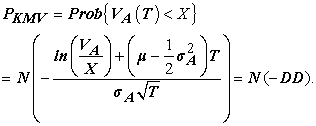Then, we can obtain the frequency planned by default (Expected Default Frequency: EDF) such as: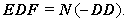However, the default probability does not correspond to the normal law. KMV Company tries to obtain the empirical value of the EDF rather than the theoretical value of the models 15. Fortunately, KMV Company possesses an enormous base of historical data concerning the default of the companies. By basing itself on these data KMV defined tables which associate with the various possible values of the distance of default (DD) on a temporal horizon considered a default probability definite and noticed empirically.

So, to protect itself against the risk which results from potential losses bound to the evolutions of the portfolio, Kealhofer, McQuown and Vasicek 16 based on the determination of a random size L relative to the losses of the portfolio which is defined in a general way and on a horizon H as follows:Where,indicates the value of the portfolio H in the absence of the losses and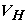indicates the market value of the portfolio H. The development follows by KMV shows us that the distribution of L can be approached by an inverse normal distribution.

4. The CreditMetrics Model

CreditMetrics was thrown for the first time in 1997 by JP Morgan's bank. CreditMetrics is considered as being an evaluation tool, for a portfolio, its variance of the values provoked by the changes of the quality of credit of the transmitter of the bonds (the credit migration) and leaves the defect of the counterpart. Unlike the approaches developed by the other models of management of a portfolio of credit, the probability of default in CreditMetrics is given by rating agencies for the big companies and by methods of scoring and mapping for small and medium-sized firms 17.

CreditMetrics belongs to the structural models since it rests on the model of Merton 3 for the definition of the thresholds of the migration of credit 4. According to Hamisultane 11, CreditMetrics makes it possible to calculate CreditVaR of a portfolio. The methodology of this model is based on the probability of moving of a quality of credit to the other in a given horizon of time (analysis of the migration of credit). The calculation of CreditVaR by CreditMetrics rests on the four stages following 2, 11:

• Determination of the risk isolated from each credit of the portfolio.

• The construction of the matrix of the probabilities of transition from a notation to another.

• The valuation of the assets of the portfolio according to the scenarios of transition from a notation to the other one.

• The calculation of CreditVaR.

The evaluation of a portfolio Value-at-Risk due to the credit (CreditVaR) by CreditMetrics is given the following Figure 2 2.

• Figure 2. The evaluation of a portfolio

In the model CreditMetrics, there are three categories of estimation to be used according to the nature of the composition of the portfolio. We are going to try, in what follows, to present the various principles of the model according to the composition of the portfolio.

4.1. The Portfolio in an Obligation

According to Hamisultane 11, the system of rating used by CreditMetrics is the one rating agency. So, the broadcasting issuers of debt securities are noted according to a ladder of seven categories going from AAA to CCC according to the financial solidity of every company 1. The notation AAA is tuned to the healthy companies financially whereas those who are characterized by a bad financial situation are noted by CCC.

The notations offered by the agencies of rating are regularly published. These notations present information relative to the broadcasting issuers of debt securities. The agencies of rating include these notations in indicating tables, either the rate of historic default of broadcasting issuers according to their notation on a horizon of well determined time, or the evolutions of these notations in the time. These tables recapitulating the notations tuned to the broadcasting issuers of debt securities are defined by "the matrices of transition".

The matrices of annual transition summarize all the changes of notation, on a horizon of time of one year, of a sand of broadcasting issuers is presented as follows (Table 3).

According to Grundke 18, this table must be carefully analyzed. So, by taking as an example the line corresponding to the BBB rating presented in Table 3, we can deduct the probability of default as follows (Table 4).

After a period of one year, and settling on the asset of initial notation BBB, we can deduct that the probability that this active rest BBB after a period of one year is 86,93 %, that to become AAA is 0,02 % and that to be lacking is 0,18 %.

The use of this model is based on three main hypotheses 11, 18, 19:

• The absence of multiple transitions: for a horizon of time given the number of transitions is in most of a single transition.

• The stability of the matrix of transition in time: for every class of notation, two companies in different sectors or in different countries have the same probability to migrate from a notation to the other one.

• The matrix of transition is of type Markov: for period given the probability to migrate of a class of notation in another class is independent from what took place for the last periods. These hypotheses are emitted for the simplification of the calculations of the matrix of transition for the posterior periods.

CreditMetrics determines the current value of the bond by using the curve of the rates with zero coupons to proceed with the calculations of CreditVaR. In that case, the transmitter of debt securities is not in situation of bankruptcy. By continuing in the same context of analysis, that is the use of the notation BBB as the example, we can use the table of the Forward rates following (Table 5).

We suppose in our case which a noted transmitter BBB has emitted a Bond for 100 Euro over 4 years with a rate without annual risk of 6 %. The current value of the bond is given by the equation below: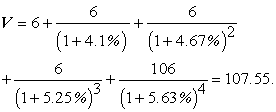By basing itself on the formula above, being able to us determine the various possible values of fire of type BBB according to his possible migrations towards other notations 1, 5. The possible values of a bond rated BBB according to the possible migrations are presented in the Table 5.

In case the company had a bankruptcy, the value of the bond is determined by using the average recovery ratio calculated by CreditMetrics on historical data 20.

Further to the representative table of the various values of BBB according to the possible migrations, we can subtract the distribution of the variations of the price of the obligation in Table 5.

The analysis of this table shows that CreditVaR in 1 % (at a level of 99 % confidence) is equal to the last value of the variation of the value of the bond which corresponds to the notation CCC. Thus, CreditVaR is equal to -23.91.

4.2. The Portfolio in Two Obligations

In the case of a portfolio consisted of two bands, the analysis is based on the level of correlation of the migrations. In fact, in a portfolio consisted of several assets the migrations of the various credits are correlated. CreditMetrics tries to estimate these correlations. As long, as there are no good data to be employed. In this case, CreditMetrics used the correlations between the values of the assets of the broadcasting issuers of the credits which are approached by the correlations among the equity prices of these broadcasting issuers to calculate the correlations between the migrations of the credits 21, 22.

Then, to be able to divert the correlations of the migrations of the credits of the correlations of the values of assets, it is necessary to have a model linking the quality of a credit to the value of assets. The solution proposed by CreditMetrics is to use an extension of the model of Merton 3 which incorporates the migrations of the credits. In this alignment, we suggest taking into account the probability of migration of a bond rated initially by BB. These probabilities are given by Table 7.

By basing itself on the model of Merton 3, we can suppose that the efficiency on a bond modeled as follows:With,a term of error is such as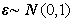,is the average efficiency on the bond andis the standard deviation of the efficiencies of this bond. Then, the default probability of an issuer of the bond is given by the following expression:Thus,If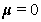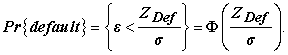Where,indicates the cumulative function of the normal law.

By using the table above, we can establish the table according to who summarizes the distribution of the probability of migration affected in conformance with BB rating:

With,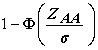represent the probability so that the bond of BB rating can pass in the notation AAA and ZAA indicates the threshold from which BB passes to AAA.

The transformation graphic of the data above is presented as follows:

• Figure 3. Generalization of the Merton model to include rating changes 

Thus,The values of the other thresholds are calculated according to whom corresponds itself aside type of the normal distribution of the random on the assets of the notation BB 2, 23, 24, 25, 26.

We suppose now, that a second issuer presents a rating A where the random on assets follow a normal distribution with a parameter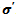. In that case, the values of thresholds relative for two bands who rated BB and A are presented as follows:

By taking into account the table above, we can calculate the probability of migration joined in the following way:With, r and r’ indicate respectively the random on the assets who are rated by BB and A andrepresent the joint density function by the Gaussian distribution which depends on the coefficient of correlation ρ.

The joint density function of the Gaussian distribution of two variables X and Y is presented by the form below: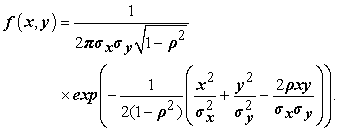According to Hamisultane 11, for ρ = 20% the matrix of joint transition which considers the correlation banding both entities BB and A is the following one:

The last column of the table and the last line of this one represent the marginal probability for the entities BB and A which are equal to the sum of the joint probability by line or by the column. According to Crouhy and al. 2 these marginal probabilities correspond to the probability of migration of BB and of A taken individually. The variation of the portfolio of both bands is calculated for each of the joint probability 27, 28.

4.3. The Portfolio in Several Obligations

In case the portfolio consists further more than 2 bands calculates its joint probability will more be complicated. So, model CreditMetrics propose the use of the simulations of Monte Carlo and the decomposition of Cholesky to generate trajectories correlated to the bond and build the distribution of the values of the portfolio on certain horizon of time 2, 29.

According to Hamisultane 11 and Feng et al. 26, to generate trajectories correlated to the variables which follow a normal distribution N (µ, ∑). The determination of these trajectories requires the respect for the following five stages:

Stage 1: The regression of the random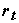of the band on the sectorial indications. For example, in the case of three bands and two sectorial indications.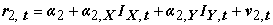To pass in the second stage it is necessary to estimate the various parameters of three models.

Stage 2: The calculation of the variances and the covariance’s banding 2 bands i and j: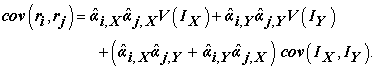And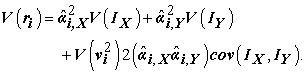By using these two formulae, we can obtain the matrix of the variances-covariance’s ∑.

Stage 3: The decomposition of Cholesky of the matrix of the variances of the variances-covariance’s ∑ in the following way 5:With A represent the lower triangular matrix and AT transposed by the matrix A.

Stage 4: The simulation of variables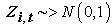. In fact, the existence of the bond to be feigned allows the existence of.

Stage 5: The simulation of the values of the correlated variables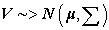by basing itself on a process of geometrical distribution: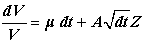Thus: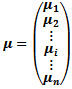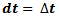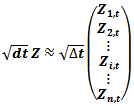According to Crouhy et al. 2, Nickell et al. 24 and Bangia et al. 25, the forces and the weaknesses of this model are presented in the following table:

5. Conclusion

In this paper we developed a comparative theoretical approach’s concerning the model of management of credit portfolio. Then, we studied the four mains models of credit portfolio management. In the financial literature those models are grouped by three types of credit portfolio models 1. The structural models: there are two models of management of credit portfolio who are supplied in the literature: Moody's KMV model (Portfolio Model) and CreditMetrics model by JPMorgan.

The KMV model and Credit Portfolio View base their approach on the same empirical observation that default and migration probabilities vary over time. The KMV model adopts a microeconomic approach which relates the probability of default of any obligor, to the market value of its assets.

References

  Koyluoglu, H., and Hickman, A.(1998). Reconcilable differences, Risk, 11(10), 56-62. In article  Crouhy, M., Galai, D. and Mark, R. (2000). A comparative analysis of current credit risk models. Journal of Banking & Finance, (24): 59-117. In article View Article  Merton, R. (1974). On the pricing of corporate debts: the risk structure of interest rates. Journal of Finance, (29): 449-470. In article View Article  Leland, H.E. (1994). Corporate Debt Value, Bond Covenants, and Optimal Capital Structure. Journal of Finance, 49, 1213-1252. In article View Article  Leland, H.E. and Toft, K.B. (1996). Optimal Capital Structure, Endogenous Bankruptcy, and the Term Structure of Credit Spreads. The Journal of Finance, 51, 987-1019. In article View Article  Ronald W. Anderson, Suresh Sundaresan; Design and Valuation of Debt Contracts, The Review of Financial Studies, Volume 9, Issue 1, Pages 37-68. In article View Article  Jarrow. R.A. (2011). Credit market equilibrium theory and evidence: Revisiting the structural versus reduced form credit risk model debate. Finance Research Letters, (8):2-7. In article View Article  EOM, Y. H., J. HELWEGE, and J. Z. HUANG (2004).Structrual Models of Corporate Bond Pricing: An Empirical Analysis, Review of Financial Studies. 17, 499-544. In article View Article  Ericsson, J., and J. Reneby, (2005). Estimating Structural Bond Pricing Models, Journal of Business, 78(2), 707-735. In article View Article  Schaefer, S.M, and Strebulaev, I. (2008). Structural Models of Credit Risk are Useful: Evidencefrom Hedge Ratios on Corporate Bonds. Journal of Financial Economics, 90(1), 1-19. In article View Article  Hamisultane, H. (2008). Modèles de gestion du risque de crédit. Investment System R&D, Document n°1. In article  Chen, N.-F., Roll, R., Ross, S.A., (1986). Economic Forces and the Stock Market, Journal of Business 59, p. 383-403. In article View Article  Black, F., and Scholes, M., (1973). The Pricing of Options and Corporate Liabilities. Journal of Political Economy, 637-654. In article View Article  Huang, S.J. and Yu, J. (2010). Bayesian analysis of structural credit risk models with microstructure noises. Journal of Economic Dynamics & Control, (34):2259-2272. In article View Article  Zhou, C., (2001). An Analysis of Default Correlations and Multiple Defaults, Review of Financial Studies, Vol. 14, S. 555-576. In article View Article  Kealhofer, S. (1993). Portfolio management of default risk, KMV Corporation. In article View Article  Paleologo, G., Elisseeff, A., and Antonini, G. (2010). Subagging for credit scoring models. European Journal of Operational Research, 201, 490-499. In article View Article  Grundke, P. (2009). Importance sampling for integrated market and credit portfolio models. European Journal of Operational Research, (194): 206-226. In article View Article  Figlewski, S., Frydman, H. and Liang, W. (2012). Modeling the effect of macroeconomic factors on corporate default and credit rating transitions. International Review of Economics and Finance, (21): 87-105. In article View Article  Gordy, M.B., 2000. A Comparative Anatomy of Credit Risk Models. Journal of Banking and Finance 24, 119-149. In article View Article  Treacy, W.F. and M. Carey, 2000, “Credit Risk Rating Systems at Large US Banks,” Journal of Banking & Finance 24, 167-201. In article View Article  Altman, E. I. and H. A. Rajken, (2004). How rating agencies achieve rating stability. Journal of Banking & Finance 28 (11), 2679-2714. In article View Article  Gupton, G.M., Finger, C.C. and Bhatia, M. (1997). CreditMetricsTM – Technical Document’, Morgan Guaranty Trust Company. In article  Nickell, P, W. Perraudin and S. Varotto, (2000). Stability of Rating Transitions, Journal of Banking & Finance 24, 203-227. In article View Article  Bangia, A., F.X. Diebold, A. Kronimus, C. Schagen and T. Schuermann, (2002). Ratings Migration and the Business Cycle, With Applications to Credit Portfolio Stress Testing, Journal of Banking & Finance 26, 445-474. In article View Article  Feng, D., C. Gourieroux, and J. Jasiak, (2008). The ordered qualitative model for credit rating transitions. Journal of Empirical Finance, 15 (1), 111-130. In article View Article  Brady, B., and R., Bos, (2002). Record Defaults in 2001. The Results of Poor Credit Quality and a Weak Economy", Special Report, Standard & Poor’s, February. In article  Brady, B., Vazza, D., and R., Bos, (2003). Ratings Performance 2002: Default, Transition, Recovery and Spreads", Website of Standard & Poor’s. In article  Fishman, G., (1997). Monte Carlo: Concepts, Algorithms, and Applications. Springer Series in Operations Research. Springer, Berlin. In article View Article  Bharath, S. and Shumay, T. (2008). Forecasting Default with the Merton Distance to Default Model. Review of Financial Studies, 21, 1339-1369. In article View Article  McQuown, J.A. (1993). Market vs. accounting based measures of default risk, KMV Corporation. In article  Vasicek, O.A. (1984). The philosophy of credit valuation: the credit valuation model, KMV Corporation. In article PubMed  Crosbie, P., (1998). Modeling Default Risk. KMV Corporation, San Francisco. In articleThis work is licensed under a Creative Commons Attribution 4.0 International License. To view a copy of this license, visit http://creativecommons.org/licenses/by/4.0/